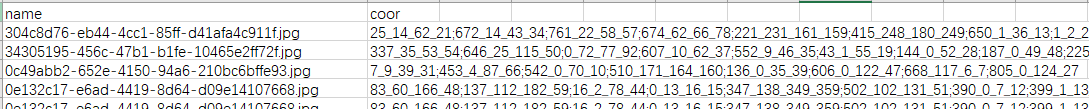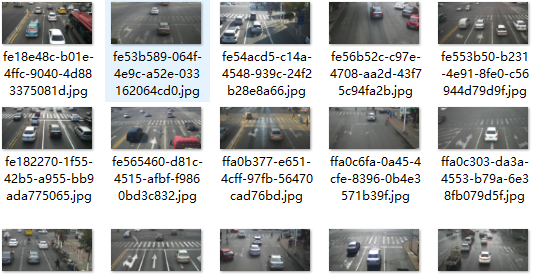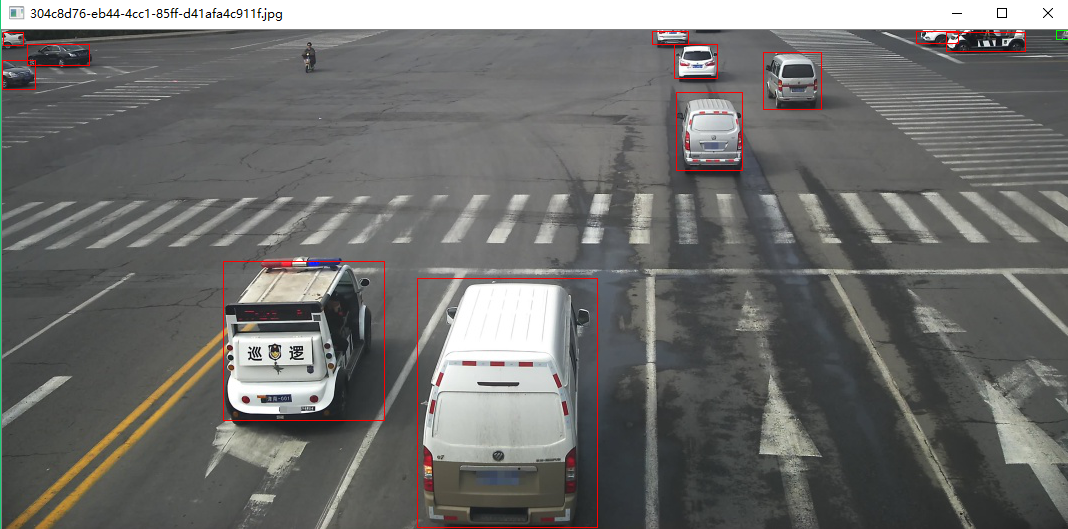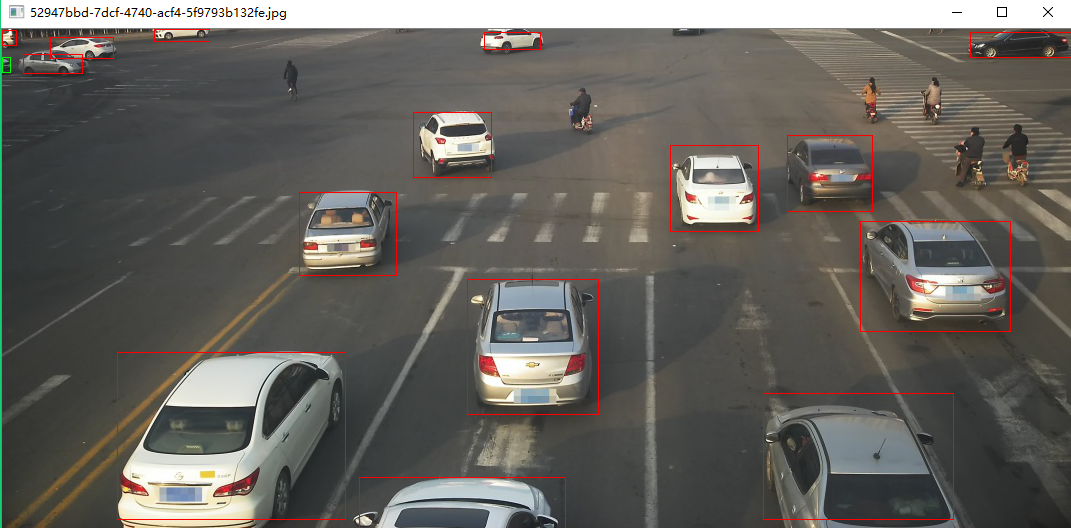# 目标检测：使用Open_cv在图像上批量画bounding box

Python 3.6.3

Open_cv 3.3.0

Numpy 1.14.2

Win10 1709教育版

## 2.数据格式

本次主要包含如下两个文件：

a.包含坐标的CSV文件，其中包含两列，一列是图片的名称，一列是图片上被检测物体的坐标，坐标表示为（x,y,w,h）；具体如下：b.被检测图片：## 3.导入Bounding Box

import numpy as np
import cv2

with open('a.csv', 'r') as p:
lines = p.readlines()[1:]

由于，csv文件里面的坐标是多个拼接起来成了一个字符串，而opcv_cv需要的是数字坐标，所以需要将这些字符串做一个分解：

for i in lines:
kk = []
rec = []
"""得到图片的路径（相对路径）"""
img_path = i.split(',')
img = cv2.imdecode(np.fromfile(img_path, dtype=np.uint8), -1)
"""得到多个矩形的坐标，分别是（x,y,w,h）"""
polygons = [r.split('_') for r in i.split(',').strip().split(';') if r]
for k in polygons:
kk.append(list(map(int, map(float, k))))
for t in kk:
rec.append(t*t)
print('{}最小'.format(kk[rec.index(min(rec))]))

## 4.使用坐标在图片上画出Bounding box

上一步我们已经拿到了一个个的坐标，接下来我们使用cv2.rectangle函数画出一个个矩形框：

    for j in kk:
if j == kk[rec.index(min(rec))]:
cv2.rectangle(img, (j, j), (j + j, j + j), (0, 255, 0))
else:
cv2.rectangle(img, (j, j), (j + j, j + j), (0, 0, 255))  

通过flags参数设定窗口的展示方式：

    cv2.namedWindow(img_path, flags=cv2.WINDOW_AUTOSIZE)
cv2.imshow(img_path, img)
cv2.waitKey(0)

## 5.附结果：